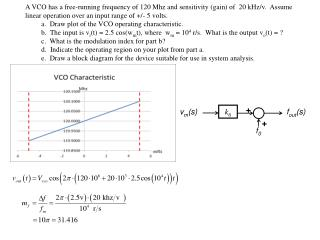# k 0 - PowerPoint PPT PresentationDownload Presentationk 0

k 0
Download Presentation## k 0

- - - - - - - - - - - - - - - - - - - - - - - - - - - E N D - - - - - - - - - - - - - - - - - - - - - - - - - - -
##### Presentation Transcript

1. A VCO has a free-running frequency of 120 Mhz and sensitivity (gain) of 20 kHz/v. Assume linear operation over an input range of +/- 5 volts. • a. Draw plot of the VCO operating characteristic. • b. The input is vi(t) = 2.5 cos(wmt), where wm = 104 r/s. What is the output vo(t) = ? • c. What is the modulation index for part b? • d. Indicate the operating region on your plot from part a. • e. Draw a block diagram for the device suitable for use in system analysis. + vm(s) k0 fout(s) + f0

2. + kd vout(s) fin(s) _ fd A frequency discriminator has a crossover frequency of 80 Mhz and sensitivity (gain) of 5.0 v/Mhz. a. Draw plot of the discriminator operating characteristic. b. The input is vi(t) = 2.5 cos(wmt), where wm = 0.5 x 109 r/s. What is vo(t) = ? c. Indicate the operating region on your plot from part a. (You choose, > +/- 2.1 v) d. Draw a block diagram for the device suitable for use in system analysis.

3. Phase Locked Loops Loop Filter VCO Input kF : v/rad kv : rad/s/v VCO + + kF _ + Phase Detector - + _

4. Frequency Error Phase Error - + + _ • Simple example: uncompensated loop • H(s) = 5 = kA • kF = 0.1 v/rad • ko = 60p x 103 rad/s/v • DwI = -10 khz = -20,000p rad/s F(s) is forward gain from input to point of interest. Frequency Error: F(s) = 1 Steady State Response = 0 Surprise! Phase Error: F(s) = 1/s VCO Voltage: F(s) = 0.5/s

5. Dynamic response: For step change in input frequency DwI, X(s) = DwI /s Frequency Error: F(s) = 1 Phase Error: F(s) = 1/s Hold in Range: Phase error

6. FM Application Vout(s) - + + _

7. FSK Application fFR = 3.5 khz fMARK = 2 kHz fSPACE= 4 kHz BR = 1333 Bit/s tBIT = 0.75 ms